Next: Inside-out Collapse Solution Up: Local Star Formation Process Previous: Ambipolar Diffusion   Contents

# Dynamical Collapse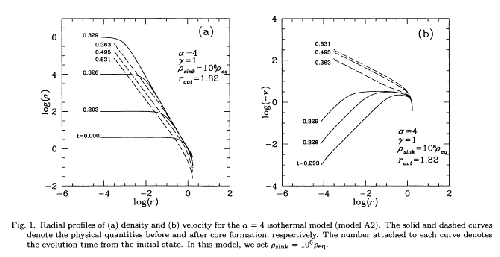In 1969, Larson (1969) and Penston (1969) found a self-similar solution which is suited for the dynamical contraction. Figure 4.5 is a radial density distribution for a spherical collapse of an isothermal cloud, where the cloud has a four-times larger mass than that of the Bonnor-Ebert mass. Although the figure is taken from a recent numerical study by Ogino et al (1999), a similar solution was obtained in Larson (1969). We can see that the solution has several characteristic points as follows:

1. The cloud evolves in a self-similar way. That is, the spatial distribution of the density (left) at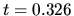is well fitted by that at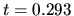after shifting in the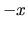and the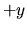directions. As for the infall velocity spatial distribution, only a shift in thedirection is needed.
2. The density distribution in the envelope, which is fitted by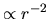, is almost unchanged. Only the central part of the cloud (high-density region) contracts.
3. The time before the core formation epoch (the core formation time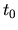is defined as the time at which the central density increases greatly) is a good indicator to know how high the central density is. That is, reading from the figure, at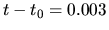() the central density reaches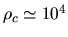and at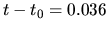() the density is equal to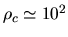. This shows the maximum (central) density is approximately proportional to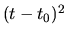, which is reasonable from the description of the free-fall time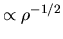.
The basic equations of spherical symmetric isothermal flow are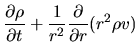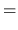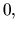(4.49)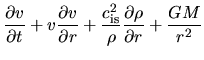(4.50)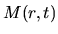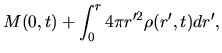(4.51)

whererepresents the mass included in the radius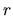and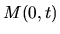denotes the mass of the protostar. A self-similar solution which has a form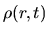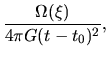(4.52)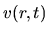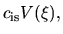(4.53)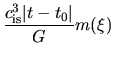(4.54)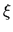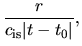(4.55)

should be found, where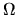and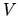are functions only on. For example, equation (4.52) asks the shape of the density distribution is the same after resizing of equation (4.55)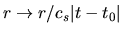and re-normalizing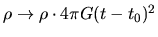as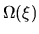. Since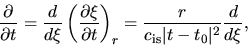(4.56)

and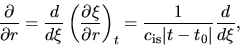(4.57)

the basic equations for the spherical symmetric model yield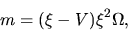(4.58)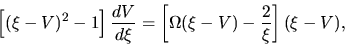(4.59)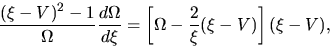(4.60)

Equations (4.59) and (4.60) have a singular point at which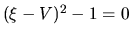or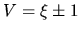. Since the point of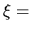const moves with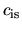, the flow velocity relative to thisconst is equal to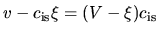. Thus the singular point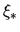at whichcorresponds to a sonic point. Therefore, since the flow has to pass the sonic point smoothly, the rhs of equations (4.59) and (4.60) have to be equal to zero at the singular point. This gives at the sonic pont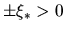,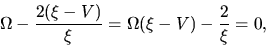(4.61)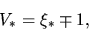(4.62)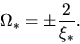(4.63)

These equations (4.58), (4.59) and (4.60) have an analytic solution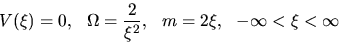(4.64)

This is a solution which agrees with the Chandrasekhar's SIS. Generally, solutions are obtained only by numerical integration.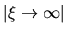the solution have to converge to an asymptotic form of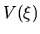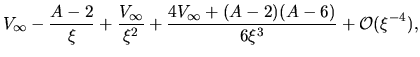(4.65)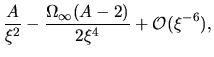(4.66)

This shows that for sufficiently large radius the gas flows with a constant inflow velocity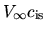.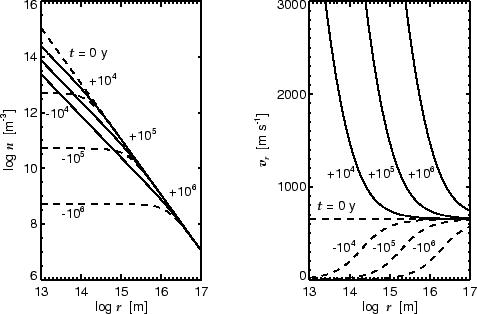This has a solution in which the density and the infall velocity should be regular with reaching the center (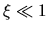). Such kind of solution is plotted in Figure 4.6 (left) and Figure 4.6 (right) with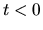. This time evolution is expected from the self-similar solution. This shows that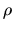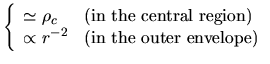(4.67)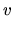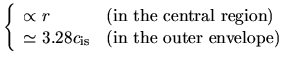(4.68)

Reaching the outer boundary the numerical solution (Fig.4.5) differs from the self-similar solution (Fig.4.6). For example,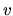is reduced to zero in the numerical simulations, while it reaches a finite value 3.28 in the self-similar solution. And as for the density distribution,drops near the outer boundary in the numerical simulations while it decreases proportional to. However, in the region except for the vicinity of the outer boundary the self-similar solution expresses well the dynamical collapse of the spherical isothermal cloud. This solution gives the evolution of a pre-protostellar core formed in a supercritical cloud/cloud core.

SubsectionsNext: Inside-out Collapse Solution Up: Local Star Formation Process Previous: Ambipolar Diffusion   Contents
Kohji Tomisaka 2007-07-08